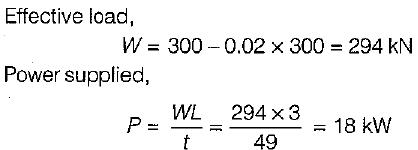Courses

# Test: Energy Transfer - 1

## 10 Questions MCQ Test Topicwise Question Bank for Mechanical Engineering | Test: Energy Transfer - 1

Description
This mock test of Test: Energy Transfer - 1 for Mechanical Engineering helps you for every Mechanical Engineering entrance exam. This contains 10 Multiple Choice Questions for Mechanical Engineering Test: Energy Transfer - 1 (mcq) to study with solutions a complete question bank. The solved questions answers in this Test: Energy Transfer - 1 quiz give you a good mix of easy questions and tough questions. Mechanical Engineering students definitely take this Test: Energy Transfer - 1 exercise for a better result in the exam. You can find other Test: Energy Transfer - 1 extra questions, long questions & short questions for Mechanical Engineering on EduRev as well by searching above.
QUESTION: 1

### Capacity of hydraulic accumulator is generally specified as

Solution:

The hydraulic accumulator is a device which is used for temporarily storing or accumulating the liquid under pressure supplied by the pump when it is not required by the machines. The maximum amount of energy that the accumulator can store is known as the capacity of the accumulator.

QUESTION: 2

### Pressure intensifier increases the pressure in proportion to

Solution:

Let suffixes 1 and 2 denote the larger and smaller cylinders respectively.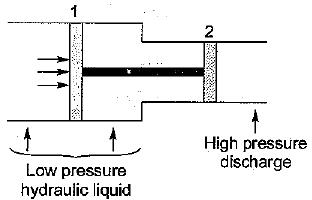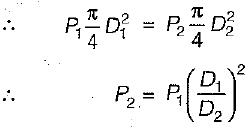Thus the input pressure is increased by a factor which is equal to the square ratio of the inlet to outlet diameter.

QUESTION: 3

### Hydraulic accumulator is used for

Solution:
QUESTION: 4

Maximum impulse will be developed in hydraulic ram when

Solution:

Impulse is created in very short duration of time. The flow of water is maximum in the supply pipe just before the closure of the waste valve.

QUESTION: 5

The pump to be used for pumping highly viscous fluids belongs to the category of

Solution:
QUESTION: 6

Consider the following statements pertaining to
hydraulic ram:
1. Hydraulic ram does not need external power.
2. It works on the fundamental priciple of water hammer.
3. The efficiency of a hydraulic ram is only of the order of 8 to 10%.
4. It can be termed as a low, intermitted discharge low-head pumping installation.

Which of the above statements are valid?

Solution:
QUESTION: 7

The energy transfer device which is used for lifting water from a well by utilizing the compressed air is known as

Solution:
QUESTION: 8

Hydraulic ram is a pump which works on the principle of

Solution:
QUESTION: 9

A jet pump is located at 3 m above the sump water level and has a head of 13 m applied at the nozzle. If the delivery head is 11 m when the discharge through the pump is 15 L/s and jet discharge is 2.5 L/s, what is the efficiency of jet pump?

Solution:

A jet pump is a combination of normal centrifugal pump and a jet device at the suction end, When the pump is started, a part of the water from delivery side of pump is diverted into a nozzle. The efficiency of jet pump is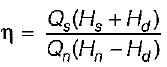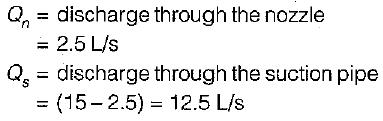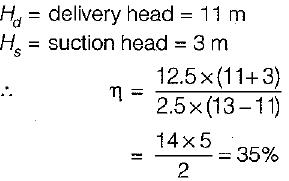QUESTION: 10

An accumulator has a ram of 250 mm in diameter. The effective stroke of the ram is 3 m and the total load on the ram is 300 kN. The frictional effects in the accumulator can be taken as 2% of the total load on the ram. What is the total power delivered by the accumulator when ram falls through its full stroke in 49 seconds?

Solution: